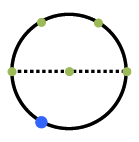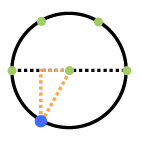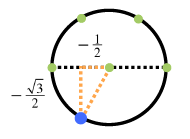### Home > PC3 > Chapter 2 > Lesson 2.2.2 > Problem2-64

2-64.

Evaluate each of the following expressions by using special right triangles and the unit circle (give exact values).1. $\sin\left(\frac{4\pi}{3}\right)$

1. Draw a unit circle and locate the angle. Circle with dashed, horizontal diameter, 5 green points located as follows: on left & right ends of diameter, at center of circle, & 2 points equally spaced, on top half. Blue point on the lower left quarter of the circle.

2. Draw a right triangle with the base is the x-axis.
Added: right triangle in fourth quadrant: horizontal leg, on the negative, x axis, hypotenuse, from center of circle to blue point.

3. Label the side lengths. Labels added: horizontal leg, negative 1 half, vertical leg, negative 1 half times square root of 3.

4. Choose the y-value for the sine!2. $\cos\left(\frac{11\pi}{3}\right)$

3. $\sin\left(-\frac{5\pi}{6}\right)$

4. $\cos\left(-\frac{2\pi}{3}\right)$